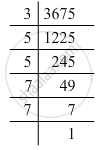Advertisement Remove all ads

# By What Number Should Each of the Following Numbers Be Multiplied to Get a Perfect Square ? Also, Find the Number Whose Square is the New Number. 3675 - Mathematics

By what number should each of the following numbers be multiplied to get a perfect square ? Also, find the number whose square is the new number.

3675

Advertisement Remove all ads

#### Solution

3675 = 3 x 5 x 5 x 7 x 7Grouping them into pairs of equal factors:
3675 = (5 x 5) x (7 x 7) x 3
The factor, 3 is not paired. For a number to be a perfect square, each prime factor has to be paired. Hence, 3675 must be multiplied by 3 for it to be a perfect square.
The new number would be (5 x 5) x (7 x 7) x (3 x 3).
Furthermore, we have:
(5 x 5) x (7 x 7) x (3 x 3) = (3 x 5 x 7) x (3 x 5 x 7)
Hence, the number whose square is the new number is:
3 x 5 x 7 = 105

Is there an error in this question or solution?
Advertisement Remove all ads

#### APPEARS IN

RD Sharma Class 8 Maths
Chapter 3 Squares and Square Roots
Exercise 3.1 | Q 7.2 | Page 4
Advertisement Remove all ads

#### Video TutorialsVIEW ALL 

Advertisement Remove all ads
Share
Notifications

View all notifications

Forgot password?
Course